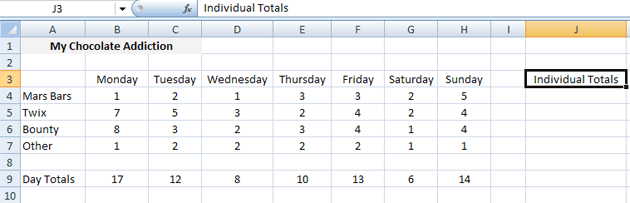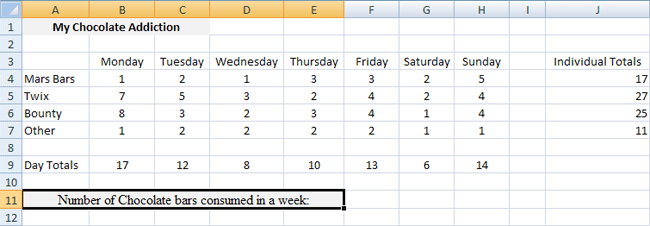Home and Learn: Microsoft Excel Course

# The SUM Function Continued

Using the same spreadsheet you've been working on in the previous section, you'll now get some more practice with the SUM function in Excel, in order to add up values in cells. Our spreadsheet now looks like this, though:You've just used the easy way to add up values in consecutive cells for a column. Just do this:

=SUM(B4:B7)

Using that formula gave us the answer to how many chocolate bars we ate from Monday to Sunday. You can use this same colon ( : ) shorthand to add up numbers in a Row.

• Type the text Individual Totals (you may have to widen the column a bit, as you did for a previous section)
•We'll use a SUM formula to add up the values in each Row. This will tell us how many of a particular chocolate bar we ate in one week: how many Mars Bars, how many Twix, etc.

The first answer we'll try is how many Mars Bars we ate in one week. We'll place this answer in cell J4. The cells we're going to be adding up are these:

B4 + C4 + D4 + E4 + F4 + G4 + H4

Because we have consecutive cells, we can use the colon shorthand again.

• Then click into the formula bar at the top
• Enter the following formula:
• =Sum(B4:H4)

Press the enter key on your keyboard, and you'll see the answer appear in J4. To complete the rest of the rows, we can use AutoFill again.

• Click back in cell J4 to make it the active cell
• Move your mouse pointer to the bottom right of cell J4
• You'll see the pointer change to a thin black cross:
•• Now hold down your left mouse button
• Keep the left button held down and drag down to cell J7
•When your mouse pointer gets to cell J7, let go of the left button. Excel will use AutoFill to get the answers for the other three cells. Hopefully, your spreadsheet now looks like ours:Select any of the cells J4, J5, J6 and J7. Then examine the formula in the formula bar. You should be able to understand what is being added up, and what all the formulas mean.

Now that we have totals for each individual chocolate bar, we can work out how many chocolate bars we ate for the whole week. We'll put the Grand Total in cell F11. First, we'll enter some text to explain what is being added up

• Type the following text: Number of Chocolate bars consumed in a week
• Hit the Enter key on your keyboard
• You should see the text you just typed. But it will all be in individual cells. Highlight the cells A11 to E11, and merge them together (You learned how to merge cells in a previous section.)
•There are two ways we can calculate the Grand Total. You can just add up the Individual totals in the J column, or ... Well, how else could you get the number of chocolate bars consumed in one week?